## Section26.2Entropy

We will examine two reversible processes for a gas in the $(p,V)$ plane as shown in Figure 26.2.1. This will help us discover a state property which will be called entropy. In Figure 26.2.1, two paths ACB and ADB are two ways to transform the gas from state A to state B.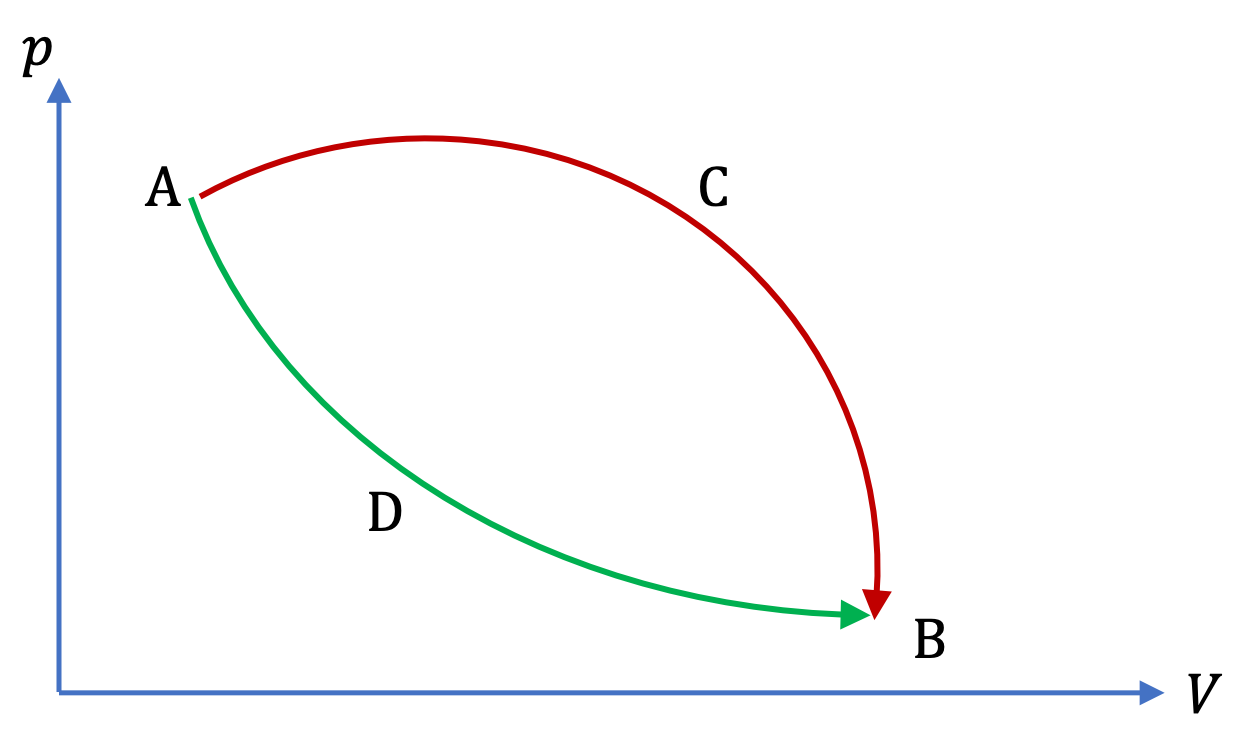Figure 26.2.1. Two reversible processes ACB and ADB that take the system from state A to state B. Since the ACBDA is a cyclic reversible process, $\sum \Delta Q/ T$ on paths ACB and ADB must equal so that the sum on paths ACB and BDA (the reverse of path ADB) equals zero.

In Figure 26.2.1, the path through the labeled points ACBDA is a cyclic reversible process, which is just ACB and revrsed-ADB. Clausius showed that we can think of the entire cyclic process ACBDA as made up of infinitely many reversible steps. We compute $\Delta Q/T$ in each step. Summing them gives the following important result.

\begin{equation*} \left( \sum \dfrac{\Delta Q}{T} \right)_{\text{cycle ACBDA}} = 0. \end{equation*}

From the way this sum is computed, it is clear that the sum is sum over two paths, ACB and BDA paths.

\begin{equation} \left( \sum \dfrac{\Delta Q}{T} \right)_{\text{cycle path}} + \left( \sum \dfrac{\Delta Q}{T} \right)_{\text{path BDA}} = 0.\label{eq-dq-t-in-cycle}\tag{26.2.1} \end{equation}

Because in a reversible step, each step is reversible. When we reverse a heating step, it turns into a cooling step, meaning if $\Delta Q/T$ was positive in a step, when you reverse that step, it will be $-\Delta Q/T\text{.}$

\begin{equation*} \dfrac{\Delta Q}{T} \Longleftrightarrow - \dfrac{\Delta Q}{T}\ \ \ \text{(reversed step)} \end{equation*}

Therefore,

\begin{equation*} \left( \dfrac{\Delta Q}{T} \right)_{\text{path BDA}} = - \left(\dfrac{\Delta Q}{T} \right)_{\text{path ADB}}. \end{equation*}

Using this in Eq. (26.2.1) and rearranging we get

\begin{equation} \left( \sum \dfrac{\Delta Q}{T} \right)_{\text{path ACB}} = \left( \dfrac{\Delta Q}{T} \right)_{\text{path ADB}}.\label{eq-dq-t-in-two-paths}\tag{26.2.2} \end{equation}

This says that $\sum(\Delta Q/T)$ is independent of the way you go from A to B as long as you perform the transformation through reversible processes. Therefore, we can write this quantity as a difference of the values of some property at states A and B. We call this property entropy and denote it by letter $S\text{.}$

\begin{equation} S_\text{B} - S_\text{A} = \left( \sum \dfrac{\Delta Q}{T} \right)_{\text{any path AB}}.\tag{26.2.3} \end{equation}

We can use this to obtain entropy of state B if we know the entropy of state A as illustrated in Figure 26.2.2

Now, suppose we choose a reference state, say the state of the system at absolute zero temperature, labeled O, and assign a value of zero for $S$ this state.

\begin{equation} S_\text{O} \equiv 0.\ \ \ \text{(Third law of thermodynamics)}\tag{26.2.4} \end{equation}

This is called the third law of thermodynamics. Using reversible processes between this reference state and any arbitrary state, say state B, we can find the value fo entropy for that state by the following, not just a difference in entropy.

\begin{equation} S_\text{B} = \left( \sum \dfrac{\Delta Q}{T} \right)_{\text{any path OB}}.\tag{26.2.5} \end{equation}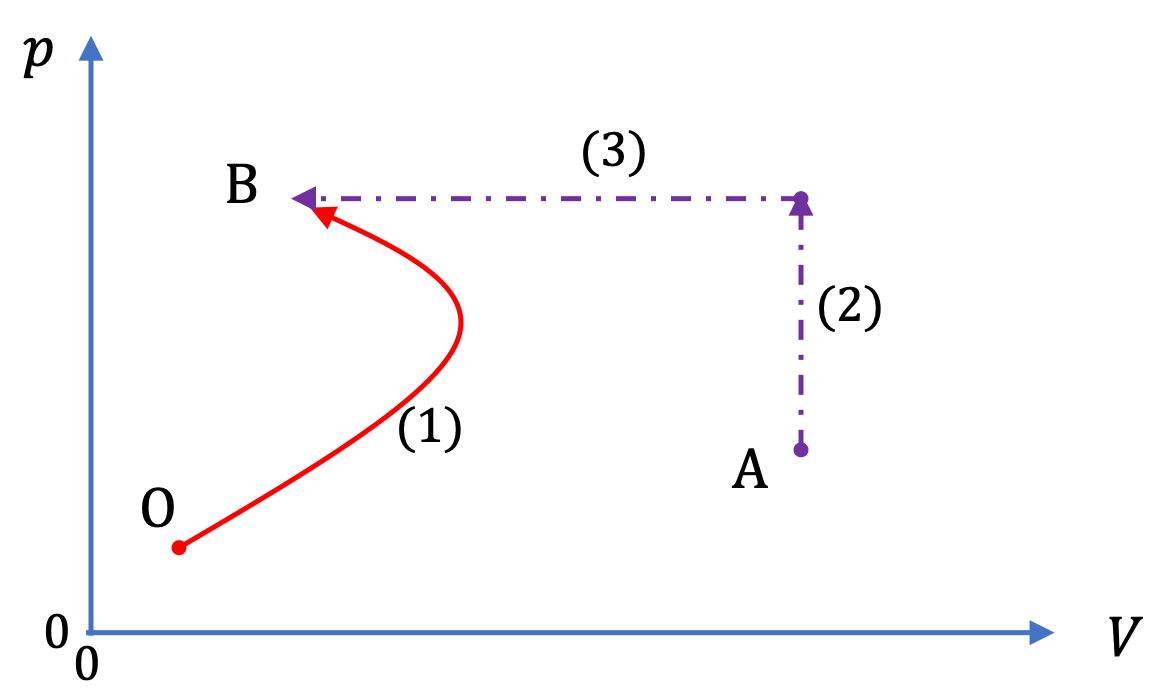Figure 26.2.2. The entropy of an arbitrary state B can be obtained in two ways. By imagining any reversible process from the standard state, shown as point O, at zero degree kelvin to the state B, shown as process (1) in the figure, we find $\sum(\Delta Q/T)$ on this path. Another way is to use a state A whose entropy is known - we imagine any reversible process from A to B and find $\sum(\Delta Q/T)$ on paths going from A to B. To that sum we add the entropy of state A to obtain the entropy of state B.

### Subsection26.2.1(Calculus) Entropy Change for an Ideal Gas in an Isochoric Process

Consider a reversible isochoric process that takes $n$ moles of a monatomic ideal gas, such as Helium, from $(P_1, T_1, V)$ to $(P_2, T_2, V)\text{.}$ Heat at constant $V$ is given by the specific heat at constant volume. We use molar specific heat $C_V$ for out calculations here.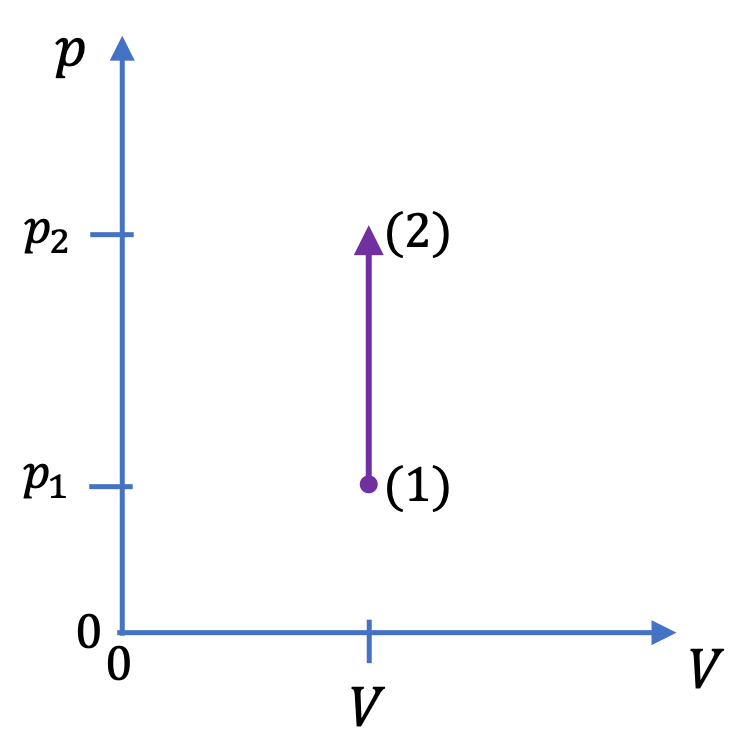The $C_V$ for a monatomic ideal gas is

\begin{equation*} C_V = \dfrac{3}{2}\,R. \end{equation*}

Therefore, heat flow in the process will be

\begin{equation*} d Q = n\, C_V\, d T = \dfrac{3}{2}n\,R\, d T. \end{equation*}

Since, we have a reversible process, we can readily write the entropy change

\begin{equation*} d S = \dfrac{d Q}{T} = \dfrac{3}{2}n\,R\, \dfrac{1}{T}\,d T. \end{equation*}

Therefore, integrating this from $T_1$ to $T_2$ gives

\begin{equation*} \Delta S = \dfrac{3}{2}n\,R\, \ln\dfrac{T_2}{T_1}, \end{equation*}

which can be rewitten by usiung the ideal gas law in terms of pressures as

\begin{equation*} \Delta S = \dfrac{3}{2}n\,R\, \ln\dfrac{P_2}{P_1}. \end{equation*}

### Subsection26.2.2(Calculus) Entropy Change for an Ideal Gas in an Isobaric Process

Consider a reversible isobaric process that takes $n$ moles of a monatomic ideal gas, such as Helium, from $(p, T_1, V_1)$ to $(p, T_2, V_2)\text{.}$ Heat at constant $P$ is given by the specific heat at constant pressure. We use molar specific heat $C_P$ for out calculations here.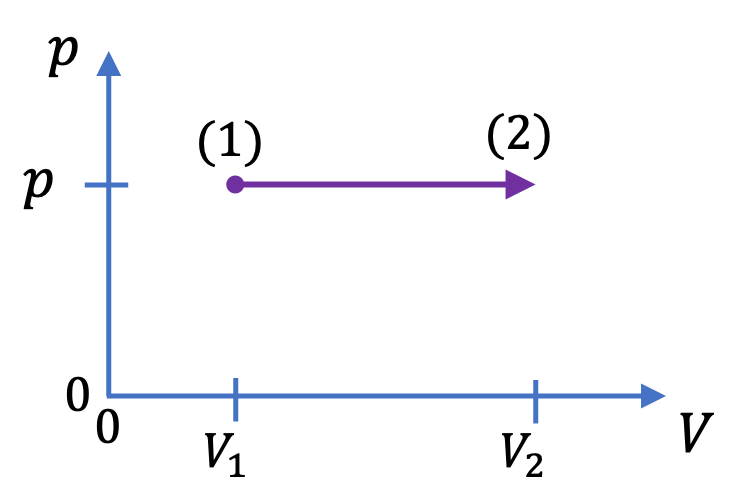The $C_P$ for a monatomic ideal gas is

\begin{equation*} C_P = C_V + R = \dfrac{5}{2}\,R. \end{equation*}

Therefore, heat flow in the process will be

\begin{equation*} d Q = n\, C_P\, d T = \dfrac{5}{2}n\,R\, dT. \end{equation*}

Since, we have a reversible process, we can readily write the entropy change

\begin{equation*} d S = \dfrac{d Q}{T} = \dfrac{5}{2}n\,R\,\dfrac{1}{T}\, dT. \end{equation*}

Therefore, integrating this from $T_1$ to $T_2$ gives

\begin{equation*} \Delta S = \dfrac{5}{2}n\,R\, \ln\dfrac{T_2}{T_1}, \end{equation*}

which can be rewitten by usiung the ideal gas law in terms of pressures as

\begin{equation*} \Delta S = \dfrac{3}{2}n\,R\, \ln\dfrac{V_2}{V_1}. \end{equation*}

### Subsection26.2.3(Calculus) Entropy Change for an Ideal Gas in an Isothermal Process

Consider a reversible isothermal process that takes $n$ moles of a monatomic ideal gas, such as Helium, from $(P_1, T, V_1)$ to $(P_2, T, V_2)\text{.}$ For an isothermal process of an ideal gas,

\begin{align*} \amp \Delta U = 0, \\ \amp W = n R T \ln \dfrac{V_2}{V_1}. \end{align*}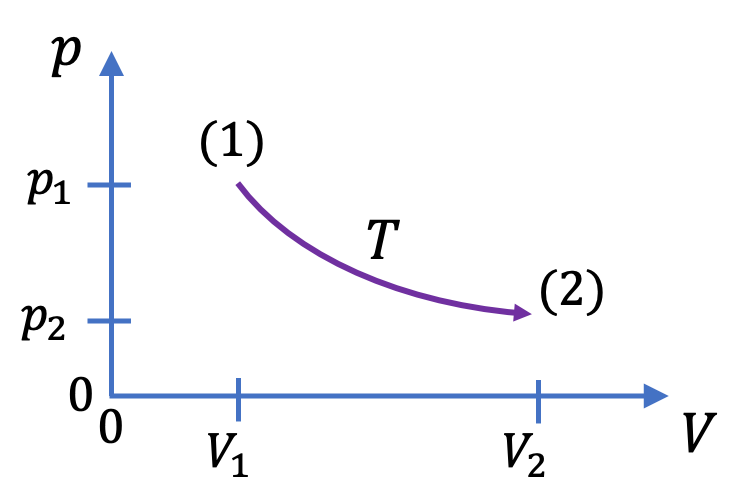The heat flow is not by specific heat anymore. Instead, we appeal to First Law of Thermodynamics for infinitesimal step, viz.,

\begin{equation*} \delta Q = dU + \delta W, \end{equation*}

Recall that $dU=0$ for isothermal process for ideal fgas, and work in an infinitesimal step is

\begin{equation*} \delta W = p dV. \end{equation*}

Therefore,

\begin{equation*} \delta Q = 0 + pdV. \end{equation*}

Therefore entropy change

\begin{equation*} dS = \dfrac{\delta Q }{T} = \dfrac{p }{T}\, dV = n R \dfrac{dV}{V}. \end{equation*}

Integrating from $V_1$ to $V_2$ we get

\begin{equation*} \Delta S = n R \ln \dfrac{V_2}{V_1}. \end{equation*}

A $50\text{-kg}$ ice block is at $0^\circ\text{C}\text{.}$ It is heated slowly melting of all ice. What is the change in entropy of the ice block?

Data: Heat of transformation of ice to liquid water = $334,000\text{ J/kg}\text{.}$

Hint

Use $Q=ml$ for the heat and then use the definition of entropy change.

$61,200\text{ J/K}.$

Solution

The heat flowing in the ice will be

\begin{equation*} \Delta Q = m l = 50\times 334,000 = 16,700,000\text{ J}. \end{equation*}

This occurs at temperature, in Kelvin scale,

\begin{equation*} T = 0 + 273 = 273\text{ K}. \end{equation*}

Therefore

\begin{equation*} \Delta S = \dfrac{\Delta Q}{T} = 61,200\text{ J/K}. \end{equation*}

Two kg water is heated reversibly from $20^{\circ}\text{C}$ to $30^{\circ}\text{C}$ at constant pressure of $1\text{ atm}\text{.}$ What is the change in entropy? Use $c_P = 1000\text{ cal/kg.K}\text{.}$

Hint

Use an infinitesimal process to set up $dQ/T$ and integrate.

$67\dfrac{\text{cal}}{\text{K}}\text{.}$

Solution

We can heat the given water infinitesimally slowly and reversibly. Heat in any infinitesimal step in which temperature is changing at a constant pressure is given by the product of mass, specific heat and temperature change.

\begin{equation*} \Delta Q = m\, c_P\, \Delta T, \end{equation*}

where $T$ is in kelvin scale. For water in the temperature range in the problem, we can assume $c_P$ to be constant. Therefore, the entropy change $\Delta S$ in one such step will be

\begin{equation*} \Delta S = \dfrac{\Delta Q}{T} = m\, c_P\, \dfrac{\Delta T}{T}. \end{equation*}

Summing up these requires Calculus. Therefore, we will write this in $dT$ rather than $\Delta T\text{.}$

\begin{equation*} d S = m\, c_P\, \dfrac{d T}{T}. \end{equation*}

Now, it is obvious that summing over the path from initial state to fiunal state will be simply an integral over temperature.

\begin{equation*} S_f - S_i = \int_{T_i}^{T_f}m\, c_P\, \dfrac{d T}{T} = m\, c_P\, \ln\left( \dfrac{T_f}{T_i}\right). \end{equation*}

Plugging in the numbers given, but making sure to use the temperatures in the kelvin scale we get

\begin{equation*} \Delta S = 2\ \text{kg} \times \dfrac{1000\ \text{cal}}{\text{kg.K}}\times \ln\left( \dfrac{303\text{K}}{293\text{K}}\right) = 67\dfrac{\text{cal}}{\text{K}}. \end{equation*}

Five moles of a monatomic ideal gas is expanded isothermally and reversibly at $100^{\circ}\text{C}$ from a volume of $10\text{ L}$ to three times the volume. What is the change in its entropy?

Hint

Use entropy change in an isothermal process discussed above.

$46\ \text{J/K}\text{.}$

Solution

We can again use the definition of entropy change we used in example above. But, this time $dQ$ is not give by specific heat definitions because temperature is constant. To find an appropriate formula for $dQ$ we will appeal to first law of thermodynamics.

\begin{equation*} dQ = dU + pdV. \end{equation*}

Since we have a monatomic ideal gas as the system, the change in internal energy is given by

\begin{equation*} dU = \frac{3}{2}nRdT. \end{equation*}

Since, we have an isothermal process, $dT=0\text{.}$ This means that $dU=0\text{.}$ Therefore, we use $dQ = pdV$ in the definition of entropy. This gives

\begin{equation*} \Delta S = \int_{V_1}^{V_2} \frac{pdV}{T}\ \ \text{(Note: integration is over volume now.)} \end{equation*}

Using the equation of state for the ideal gas we can replace $p/T$ by $nR/V\text{,}$ which can be integrated to give

\begin{equation*} \Delta S =nR\ln\left(\frac{V_2}{V_1} \right) \end{equation*}

Now, we put the numerical values to obtain the change in entropy.

\begin{equation*} \Delta S = 5\text{ mol}\times \frac{8.3\ \text{J}}{\text{mol.K}}\times \ln(3) = 46\ \text{J/K}. \end{equation*}

Evaluate the entropy change when a 5-kg block of copper is heated from $20^{\circ}\text{C}$ to $70^{\circ}\text{C}$ at the atmospheric pressure. Describe in words the thermodynamic process you have used for the calculation of the entropy change.

Data: $c_\text{Cu} = 386\ \frac{\text{J}}{\text{kg.K}}\text{.}$

Hint

Use $dQ = m c dT$ and then integrate.

$304\ \frac{\text{J}}{\text{K}}\text{.}$

Solution

Imagine the block at temperature $T$ placed in a heat bath whose temperature is only infinitesimally higher, say $T+dT\text{,}$ so that the temperature of the block rises reversibly. The block will absorb heat given by

\begin{equation*} dQ = m\, c\, dT. \end{equation*}

In this process the change in the entropy of the block will be

\begin{equation*} dS = \frac{m\, c\, dT}{T}, \end{equation*}

where $m$ is the mass of the block, $c$ the specific heat of copper, and $T$ the temperature in the Absolute scale. To obtain the entropy change over a finite process given in the question we integrate from $T_1$ to $T_2\text{.}$

\begin{align*} \Delta S \amp = \int_{T_1}^{T_2}\frac{m\, c\, dT}{T}\\ \amp = m\, c\, \ln \left( \frac{T_2}{T_1} \right). \end{align*}

Now, we will put in the numbers.

\begin{align*} \amp m = 5\ \text{kg},\ \ c = 386 \frac{\text{J}}{\text{kg.K}},\\ \amp T_1 = 293\ \text{K}, \ \ T_2 = 343\ \text{K}. \end{align*}

These give the following for the change in the entropy.

\begin{align*} \Delta S \amp = 5\ \text{kg}\times 386\ \frac{\text{J}}{\text{kg.K}}, \times\ln \left( \frac{343\ \text{K}}{293\ \text{K}} \right)\\ \amp = 304\ \frac{\text{J}}{\text{K}}. \end{align*}